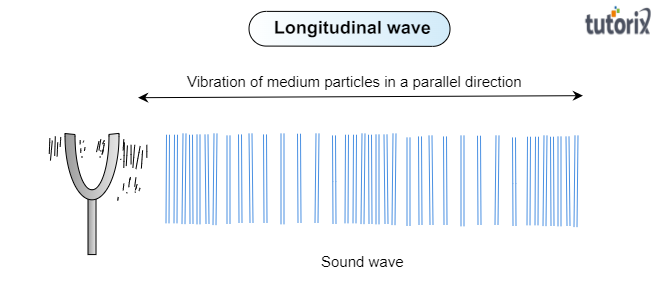# Why is a sound wave called a longitudinal wave?

To do:

To explain why a sound wave is called a longitudinal wave.

Solution:

To explain a sound wave as a longitudinal wave we should know first what are longitudinal waves and they are formed. Let us know about the longitudinal waves:

Longitudinal waves:

When the medium particles vibrate in back and forth in the same direction as the waves. these waves are called longitudinal waves. These longitudinal waves can be produced in solids, liquids, and gas.

Examples of longitudinal waves:

The following are examples of longitudinal waves-

1. Sound wave

2. Ripples on the surface of the water

Based on the definition and example of a longitudinal wave let us discuss why a sound wave is called a longitudinal wave-

Sound is a longitudinal wave:

As particles of the medium through which the sound is transported vibrate parallel to the direction that the sound wave moves, so the sound waves in the air $(or\ any\ fluid\ medium)$ are called longitudinal.

Diagram for Sound wave:

See the diagram below that shows how the medium particles move back and forth in the direction of a sound wave:Updated on: 10-Oct-2022

143 Views